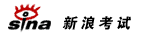# 2008年高考数学复习：解析几何专题热点指导

http://www.sina.com.cn 2008年03月07日 09:19   城市快报

天津市第四十二中学 张鼎言

5. 已知抛物线y2=2px(p>0)的焦点为F，点P1(x1，y1)，P2(x2，y2)，P3(x3，y3)在抛物线上，且2x2=x1+x3，则有( )

A. |FP1|+|FP2|=|FP3|

B. |FP1|2+|FP2|2=|FP3|2

C. 2|FP2|=|FP1|+|FP3|

D. |FP2|2=|FP1|・|FP3|

分析∵P1、P2、P3在抛物线上，

∴由抛物线定义

|PF1|=x1-(--)

=x1+-

|PF2|=x2+-

|PF3|=x3+-

又2x2=x1+x3

2(x2+-)=(x1+-)+(x3+-)

∴2|FP2|=|FP1|+|FP3|

选C

6. 已知抛物线y=-x2+3上存在关于直线x+y=0对称的相异两点A、B，则|AB|等于( )

(A)3 (B)4

(C)3- (D)4-

解：A(x1，y1)，与B(x2，y2)关于直线x+y=0对称，又A、B在抛物线上，

-

(2)-(1)：y1+x1=-x12+y12=(y1+x1)(y1-x1)

∵点A不在直线x+y=0上

∴x1+y1≠0，y1-x1=1，y1=x1+1代入(1)

-

A(-2，-1)，B(1，2)反之亦然

∴|AB|=3-，选C

7. 双曲线C1：---=1(a>0，b>0)的左准线为l，左焦点和右焦点分别为F1和F2；抛物线C2的准线为l，焦点为F2；C1与C2的一个交点为M，则---等于( )

A. -1 B. 1

C. -- D. -

解：|F1F2|=2c，设|MF1|=x，|MF2|=y

由M在双曲线C1上，x-y=2a

M在抛物线C2上，|MN|= |MF2|=y

又M在C1上，由双曲线第二定义-=-=-

-

---

=---=-1 选A

注：本题把双曲线定义、第二定义与抛物线定义连结在一起，这里M在C1、C2上是突破口，所以几何图形上的公共点是知识点的交叉点，是设计问题的重要根源.

(三) 直线与圆锥曲线相切

复习导引：学习了导数，求圆锥曲线的切线多了一条重要途径，归结起来求切线可用判别式△=0或求导.

1. 如图，在平面直角坐标系xOy中，过y轴正方向上一点C(0，c)任作一直线，与抛物线y=x2相交于A、B两点，一条垂直于x轴的直线，分别与线段AB和直线l：y=-c交于P，Q，(1)若-・■=2，求c的值；

(2)若P为线段AB的中点，求证：QA为此抛物线的切线；

(3)试问(2)的逆命题是否成立？说明理由。

解：(1)-

设A(x1，y1)、B(x2，y2)即A(x1，x12)、B(x2，x22)

△=k2+4c>0

x1+x2=k，x1・x2=-c，y1・y2=(x1・x2)2 =c2

-・■=x1x2+(x1・x2)2=c2-c=2→c=2，c=-1(舍去)

解(2)线段AB中点P(xp，yp)

xp=-，yp=-

∴xp=-，Q(-，-c)

kAQ=-

=-=2x1

又过A点的切线斜率

k=y'-=2x1

∴AQ是此抛物线在A点的切线。

解(3)过A点的切线：y-y1=2x1(x-x1)

y-x12=2x1(x-x1)

化简 y=2x1x-x12

Q(-，-c)是否满足方程。

y=2・x1・■-x12=x1・x2=-c

∴过A点的切线过Q点

∴逆命题成立

更多高考信息请访问：新浪高考频道 高考论坛 高考博客圈

特别说明：由于各方面情况的不断调整与变化，新浪网所提供的所有考试信息仅供参考，敬请考生以权威部门公布的正式信息为准。[高　考]高考进行时(音频) 直击08艺考招生
 [其　他]中西有别：英汉文化八大差异

 网页 新闻

 ・《对话城市》直播中国 ・新浪特许频道免责公告 ・诚招合作伙伴 ・企业邮箱畅通无阻